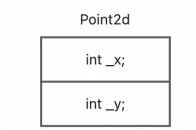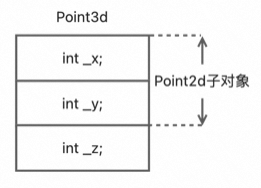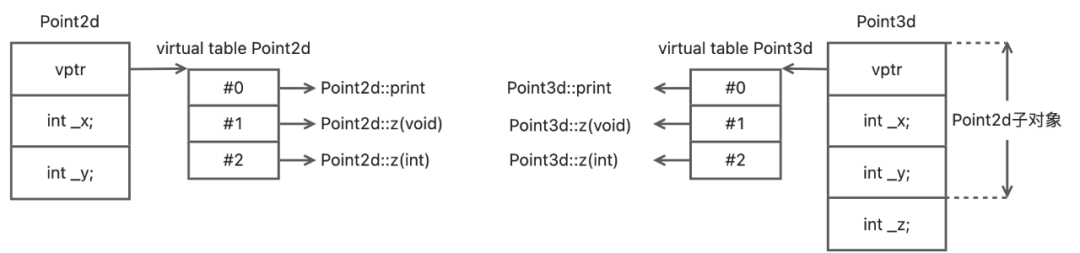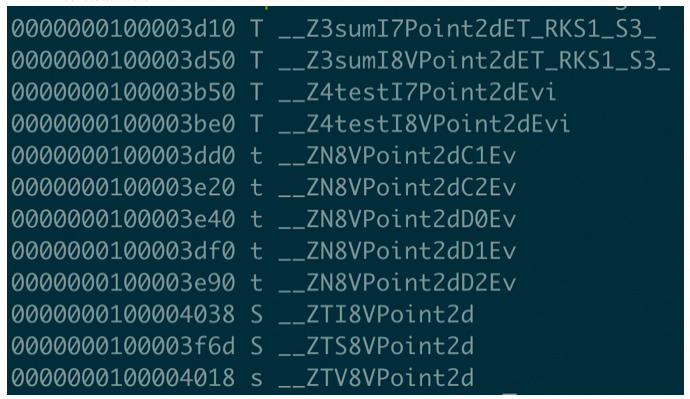Why am I writing this article? The main reason is that the code in my project uses a lot of classes with the virtual keyword, and I want to talk about it in this article. virtual doesn’t have any superpower to turn corruption into magic, it has its reasons for existence, but abusing it is a very undesirable and wrong behavior. This article will take you step by step through the virtual mechanism and unravel the mystery of virtual for you.

## Why do we need virtual

Suppose we are working on the design implementation of a public graphical library which involves printing of 2d and 3d coordinate points, and design the implementation of Point2d and Point3d as follows.

 `````` 1 2 3 4 5 6 7 8 9 10 11 12 13 14 15 16 17 18 19 20 21 22 23 `````` ``````#include class Point2d { public: Point2d(int x = 0, int y = 0): _x(x), _y(y) {} void print() const { printf("Point2d(%d, %d)\n", _x, _y); } protected: int _x; int _y; }; class Point3d : public Point2d { public: Point3d(int x = 0, int y = 0, int z = 0):Point2d(x, y), _z(z) {} void print() const { printf("Point3d(%d, %d, %d)\n", _x, _y, _z); } protected: int _z; }; int main() { Point2d point2d; Point3d point3d; point2d.print(); //outputs: Point2d(0, 0) point3d.print(); //outputs: Point3d(0, 0, 0) return 0; } ``````

Perfect, everything is as expected. If that’s the case, why do we need virtual? Let’s propose a new requirement: encapsulate a coordinate point printing interface where the input is a coordinate point instance and the output is the value of the coordinate point.

Soon, we have implemented the following code.

 `````` 1 2 3 4 5 6 7 8 9 10 11 `````` `````` void print(const Point2d &point) { point.print(); } int main() { Point2d point2d; Point3d point3d; print(point2d); //outputs: Point2d(0, 0) print(point3d); //outputs: Point2d(0, 0) return 0; } ``````

The problem is that when we pass in 3d coordinate point instances, our expectation is to print the values of 3d coordinate points, while in reality we can only print the values of 2d coordinate points. Now the program can’t tell whether the coordinate points are 2d or 3d, so in order to make the program smarter, it needs the right remedy, and `virtual` is the remedy for this problem. All that is needed is to update the declaration of the Point2d interface print.

 `````` 1 2 3 4 5 6 7 8 9 10 11 12 `````` `````` class Point2d { public: virtual void print() const { printf("Point2d(%d, %d)\n", _x, _y); } }; int main() { Point2d point2d; Point3d point3d; print(point2d); //outputs: Point2d(0, 0) print(point3d); //outputs: Point3d(0, 0, 0) return 0; } ``````

Nice job, everything is back to perfect as before. The power of implementing polymorphism in c++ inheritance relationships is exactly where `virtual` is needed. So where does its magic come from? It all starts with the memory layout of class data members.

## Memory layout of classes

In the c++ object model, non-static data members are configured within each class object, and static data members are stored outside of the class object. Static and non-static function members are also stored outside of the class object. Most compilers arrange the memory layout of classes in the order in which the members are declared. All examples in this article are compiled in the mac environment using `x86_64-apple-darwin21.6.0/clang-1300.0.29.3`, the non-virtual version of Point2d memory layout.Memory layout requires us to pay attention to the way the compiler aligns memory. Memory alignment is generally divided into two steps: one is that class members are first aligned by their own size, and the other is that the class is aligned by the size of the largest member. When we arrange the class members, we should follow the order of declaring the members from largest to smallest, so that we can avoid unnecessary memory filling and save memory occupation.

## Memory Layout of Derived Classes

In the C++ inheritance model, the memory size of a subclass is the sum of the size of its base class’s data members plus its own data members. Most compilers arrange the memory layout of subclasses with the data members of the base class first, followed by their own data members.

The non-virtual version of Point3d has the following memory layout.## The memory layout of virtual classes

When Point2d declares a virtual function, it has two major effects on the class object: First, the class will generate a series of pointers to virtual functions that are placed in a table, which is called a virtual table (vtbl). The second is that class instances are placed with a pointer to the relevant virtual table, which is usually called vptr.

For the sake of the example, we redesigned the Point2d and Point3d implementations.

 `````` 1 2 3 4 5 6 7 8 9 10 11 12 13 14 15 16 17 18 19 20 `````` `````` class Point2d { public: Point2d(int x = 0, int y = 0): _x(x), _y(y) {} virtual void print() const { printf("Point2d(%d, %d)\n", _x, _y); } virtual int z() const { printf("Point2d get z: 0\n"); return 0; } virtual void z(int z) { printf("Point2d set z: %d\n", z); } protected: int _x; int _y; }; class Point3d : public Point2d { public: Point3d(int x = 0, int y = 0, int z = 0):Point2d(x, y), _z(z) {} void print() const { printf("Point3d(%d, %d, %d)\n", _x, _y, _z); } int z() const { printf("Point3d get z: %d\n", _z); return _z; } void z(int z) { printf("Point3d set z: %d\n", z); _z = z; } protected: int _z; }; ``````

Most compilers place the vptr at the beginning of the class instance. Now let’s look at the memory layout of the virtual versions of Point2d and Point3d.Whether the real memory layout is as shown in the above diagram is very simple, we will know in a test

 `````` 1 2 3 4 5 6 7 8 9 10 11 12 13 14 15 `````` ``````int main() { typedef void (*VF1) (Point2d*); typedef void (*VF2) (Point2d*, int); Point2d point2d(11, 22); intptr_t *vtbl2d = (intptr_t*)*(intptr_t*)&point2d; ((VF1)vtbl2d)(&point2d); //outputs: Point2d(11, 22) ((VF1)vtbl2d)(&point2d); //outputs: Point2d get z: 0 ((VF2)vtbl2d)(&point2d, 33); //outputs: Point2d set z: 33 Point3d point3d(44, 55, 66); intptr_t *vtbl3d = (intptr_t*)*(intptr_t*)&point3d; ((VF1)vtbl3d)(&point3d); //outputs: Point3d(44, 55, 66) ((VF1)vtbl3d)(&point3d); //outputs: Point3d get z: 66 ((VF2)vtbl3d)(&point3d, 77); //outputs: Point3d set z: 77 return 0; } ``````

The key core virtual table is obtained in line 5, which can actually be seen as a two-step operation: `intptr_t vptr2d = *(intptr_t*)&point2d;` `intptr_t *vtbl2d = (intptr_t*)vptr2d;` the first step makes vptr2d point to the virtual table. The second step converts the pointer to the array first address. Then you can call the virtual functions one by one with vtbl2d. The output shows that the program does call the corresponding virtual functions one by one, and the memory layout of the virtual class is consistent with the structure we drew earlier.

Another interesting point is the definition of a pointer to a virtual function. You’re not wrong, it’s the existence of the c++ class `this` pointer: the `this` pointer in a class member function is actually the address of the class instance that the compiler passes in as the first argument. Like any other parameter, there is nothing special about the `this` pointer!

## virtual destructor

We didn’t even design the destructor in the previous article because we want to explain it separately here.

Let’s redesign the inheritance system and add the `Point` class.

 `````` 1 2 3 4 5 6 7 8 9 10 11 12 13 14 15 16 17 18 19 20 21 22 23 24 25 26 `````` `````` class Point { public: ~Point() { printf("~Point\n"); } }; class Point2d : public Point { public: ~Point2d() { printf("~Point2d"); } }; class Point3d : public Point2d { public: ~Point3d() { printf("~Point3d"); } }; int main() { Point *p1 = new Point(); Point *p2 = new Point2d(); Point2d *p3 = new Point2d(); Point2d *p4 = new Point3d(); Point3d *p5 = new Point3d(); delete p1; //outputs: ~Point delete p2; //outputs: ~Point delete p3; //outputs: ~Point2d~Point delete p4; //outputs: ~Point2d~Point delete p5; //outputs: ~Point3d~Point2d~Point return 0; } ``````

As you can see, in the non-virtual version of the destructor, the factor that determines the chain of destructor calls in the inheritance system is the declared type of the pointer: the destructor call starts with the class that declared the pointer type and calls its parent class destructor in order. Now let’s declare Point’s destructor as `virtual` and see the result of the same call.

 `````` 1 2 3 4 5 6 7 8 9 10 11 12 13 14 15 `````` `````` // All are unchanged except that Point destructions are declared as virtual int main() { Point *p1 = new Point(); Point *p2 = new Point2d(); Point2d *p3 = new Point2d(); Point2d *p4 = new Point3d(); Point3d *p5 = new Point3d(); delete p1; //outputs: ~Point delete p2; //outputs: ~Point2d~Point delete p3; //outputs: ~Point2d~Point delete p4; //outputs: ~Point3d~Point2d~Point delete p5; //outputs: ~Point3d~Point2d~Point return 0; } ``````

In the virtual destructor version, the factor that determines the chain of destructor calls in the inheritance system is the actual type of the pointer: the destructor calls start with the class of the actual type pointed to by the pointer and call the destructor of its parent class in turn.

## When do you need virtual?

I’ve seen a lot of modules in projects where a lot of classes declare destructors as virtual regardless of the class, and the point is that such classes are not designed for base class inheritance nor are they designed to use polymorphic capabilities, which is a crying shame. Now can you understand why it is wrong to abuse virtual? Because introducing virtual when it is not necessary is not a wise choice. It has two obvious side effects: one is an extra pointer-sized memory footprint per class, and the other is an extra layer of indirection in function calls. Both of these features will result in additional memory and performance consumption.

Among other things, memory consumption is a fixed size of a pointer, which may seem insignificant, but can introduce 100%+ memory bloat when the class has no members or few members. The performance consumption is even more insidious. virtual brings about forced synthesis of constructors, which may be unexpected to many people. Why? Because the virtual table pointer needs to be placed properly, so the compiler needs to do this at class construction time. If we were to declare another virtual destructor, we would introduce another non-essential synthesis function, causing double the performance drain. Let’s look at the consequences of this.

 `````` 1 2 3 4 5 6 7 8 9 10 11 12 13 14 15 16 17 18 19 20 21 22 23 24 25 26 27 28 29 30 31 32 `````` `````` #include #include struct Point2d { int _x, _y; }; struct VPoint2d { virtual ~VPoint2d() {} int _x, _y; }; template T sum(const T &a, const T &b) { T result; result._x = a._x + b._x; result._y = a._y + b._y; return result; } template void test(int times) { clock_t t1 = clock(); for (int i = 0; i < times; ++i) { sum(T(), T()); } clock_t t2 = clock(); printf("clocks: %lu\n", t2 - t1); } int main() { test(1000000); test(1000000); return 0; } ``````

Suppose you save the above code as `demo.cpp`, compile the code into a demo with `clang++ -o demo demo.cpp`, and use `nm demo|grep Point2d` to see all the relevant symbols.You can see that VPoint2d automatically synthesizes the constructor and destructor functions, as well as typeinfo information. As a comparison Point2d does not synthesize any function, we look at the execution efficiency of the two: on the author’s mac machine, the results of three demo executions take the middle value of Point2d: 12819, VPoint2d: 21833, VPoint2d performance time increased by 9014 clocks, an increase of 70.32%.

Therefore, be sure not to introduce virtual at will, be sure not to introduce virtual at will, be sure not to introduce virtual at will, be sure not to introduce virtual at will, unless you really need it:.

1. when using polymorphic capabilities in inheritance, you need to use the virtual functions mechanism.
2. the need to use virtual destructor functions when the base class pointer points to a subclass instance.

Any other time, virtual doesn’t have any of the other magic you want and can backfire. In fact, there is another case where virtual is needed, which is virtual base class. Since this case is too complicated, it is recommended not to try it at any time (another long article may be needed to explain why it is not recommended, so let’s leave it out of this article for now).

This is the end of the explanation of virtual, no more, no less, I don’t know if it’s enough for you. I hope this article will help you to understand and use virtual. c++ is complex and huge, and many features have their own scenarios and limitations. We only need to understand the mechanism behind it deeply to be able to do it with ease.